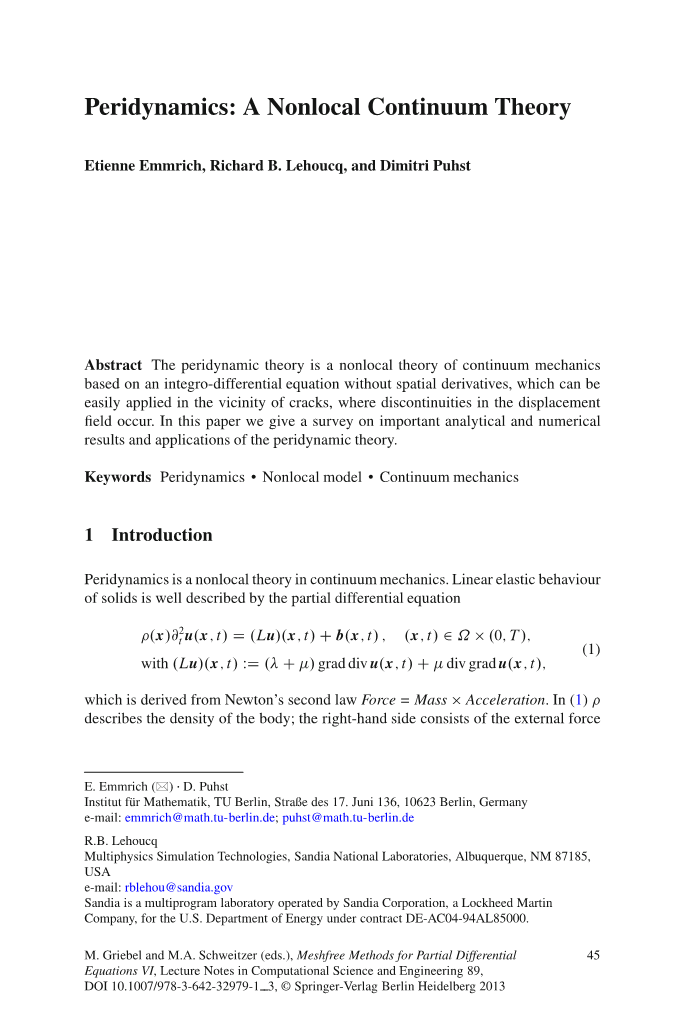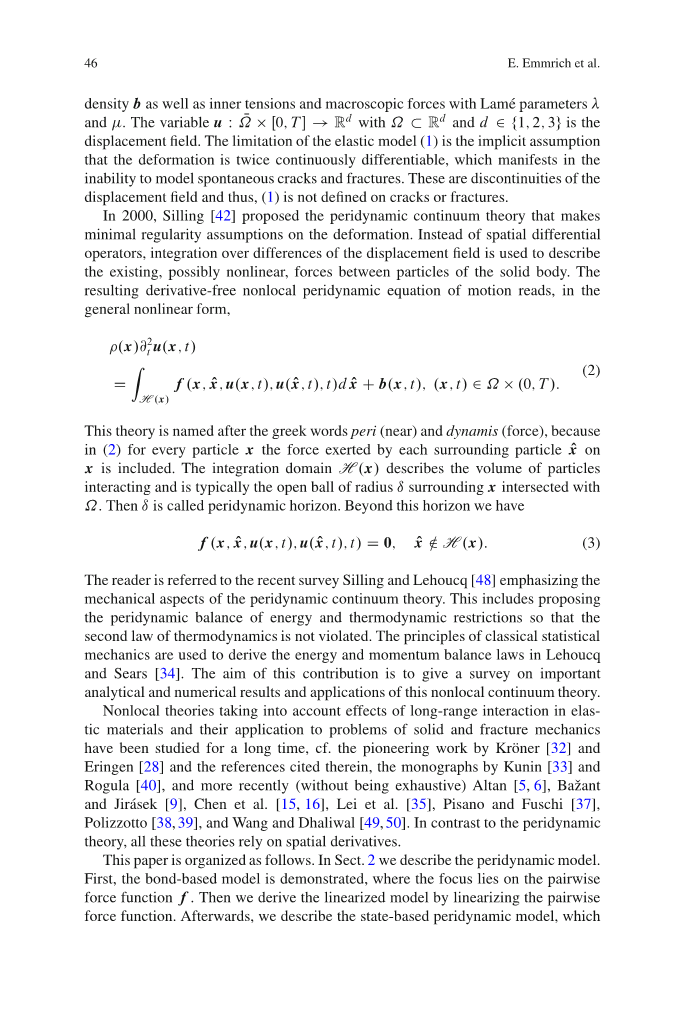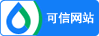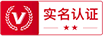### 注册

•• 获取手机验证码 60
• 注册

### 找回密码

•• 获取手机验证码60
• 找回

### 近场动力学：一种非局部连续理论外文翻译资料

2022-10-22 04:10Etienne Emmirich，Richard B.Lehoucq,和Dimitri Puhst

1. 介绍

))=(Lu)(x,t) b(x,t), (x,t)

(Lu)(x,t):=( (1)

U:with and d

2000年，Silling 基于变形最小正则性假设提出了近场动力学连续体理论，即使用离散位移场的积分来代替空间微分算子。用于描述固体本体的颗粒之间存在的与可能的非线性相互作用力。得到的不含微分项的非局部近场动力学运动方程，其一般非线性形式为，

))

=(x,u(x,t),u(,t),t)d b(x,t),(x,t) (2)

=0. (3)

2近场动力模型

2.1基于键模型

u(,0)= and u(,0)= (4)

f(,x,-=- f(x,, (5)

f (=s( （6）

s(=表示键的伸长率（bond stretch），键的伸长率是一个键长度的相对改变。我们用 || 表示欧几里得形式。在这种情况下，相关的势函数由下式给出

w(= ）

=，==

（x,t）=

（x,t）=

2.2线性化

f((

))=（）（x,t） b(x,t), （x,t）

（）(x,t):=

(r)= (r)

2.3基于态模型

e(),其中

Silling等人在文章. 中 介绍了基于物质点状态的近场动力学理论，后续推广至Silling 在文章[ 42, 15]中的方法上，公式（2）的积分被取代

)(x,t)= (8)

))=)(x,t) b(x,t),(x,t) (9)

[x,t]= (11)

[x,t]-[,t]

= f(- f(

= f(

=:

)= d (12)

-( (13)

-([]d (14)

=((15)

2.4弹性理论中的其他非局部模型

)=(g(u(,t))d, (x,t) (16)

-

Eringen  和 Polizzotto  提出和发展了Eringen模型。结合运动方程

)=div

=))d

()

3近场动力模型的数学分析

3.1的线性基于键模型

### 最新文档•